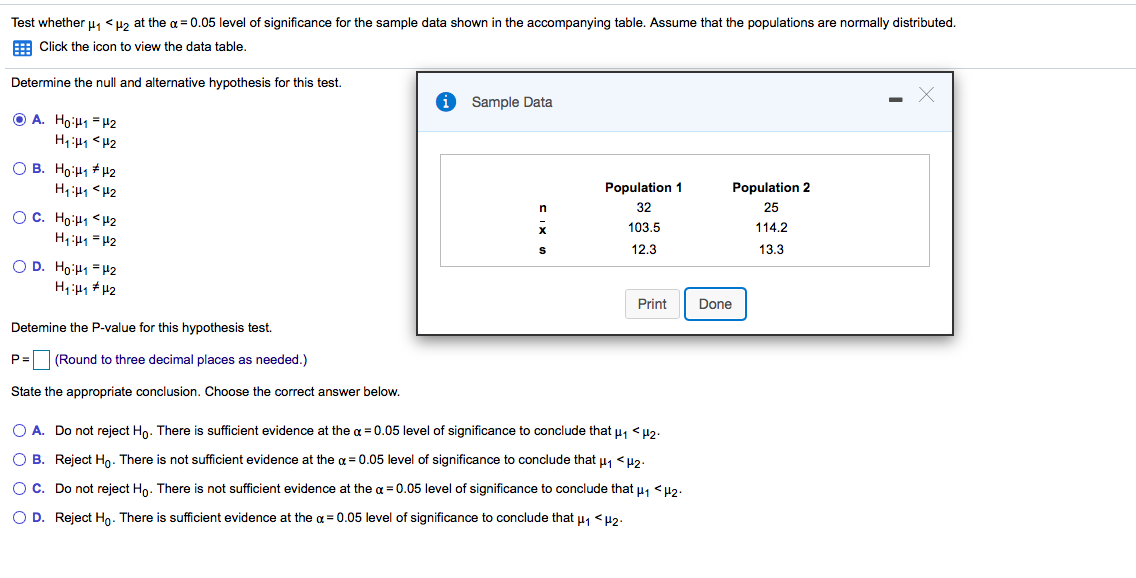# Question Test whether , <H2 at the a = 0.05 level of significance for the sample data shown in the accompanying table. Assume that the populations are normally distributed. Click the icon to view the data table. Determine the null and alternative hypothesis for this test. 0 Sample Data Population 2 O A. Ho:41 = H2 HH1 *H2 O B. Ho:H *12 H4:41 <H2 OC. HO:H1 H2 H1:41 = 2 OD. Ho:41 = 42 H1:47 *42 Population 1 32 103.5 12.3 114.2 13.3 Print Done Detemine the P-value for this hypothesis test. Pa (Round to three decimal places as needed.) State the appropriate conclusion. Choose the correct answer below. O A. Do not reject Ho. There is sufficient evidence at the a = 0.05 level of significance to conclude that 1 <H2 O B. Reject Ho. There is not sufficient evidence at the a= 0.05 level of significance to conclude that H1 H2 OC. Do not reject Ho. There is not sufficient evidence at the a = 0.05 level of significance to conclude that 11 <H2- OD. Reject Ho. There is sufficient evidence at the a = 0.05 level of significance to conclude that My <H2-AEX85B The Asker · Probability and StatisticsTranscribed Image Text: Test whether ,
More
Transcribed Image Text: Test whether ,